# 一、算法介绍

多属性决策是现代决策科学的一个重要组成部分，它的理论和方法在工程设计、经济、管理和军事等诸多领域中有着广泛的应用，如:投资决策、项目评估、维修服务、武器系统性能评定、工厂选址、投标招标、产业部门发展排序和经济效益综合评价等.
多属性决策的实质是利用已有的决策信息通过一定的方式对一组(有限个)备选方案进行排序或择优.它主要由两部分组成:
(1)获取决策信息．决策信息一般包括两个方面的内容：属性权重和属性值。
(2)通过一定的方式对决策信息进行集结并对方案进行排序和择优。信息集结方法有很多，本节只用加权算术平均(WAA)算子，就相当于计算加权平均数。

## 1. 加权算术平均算子（WAA）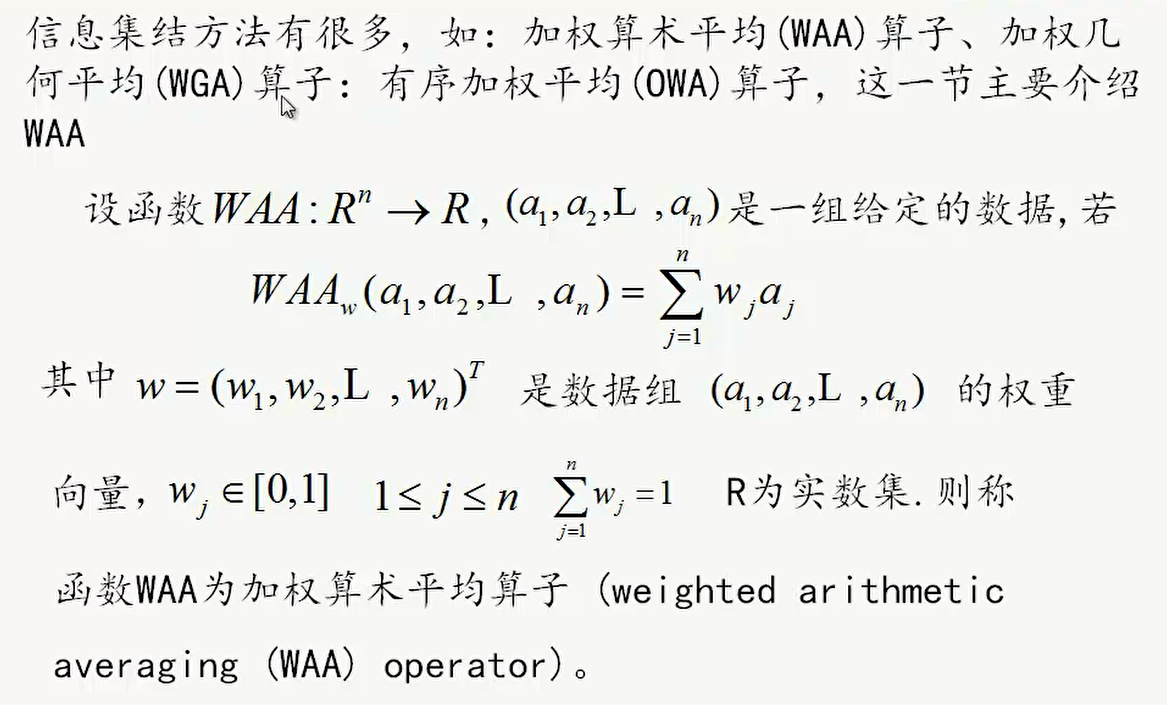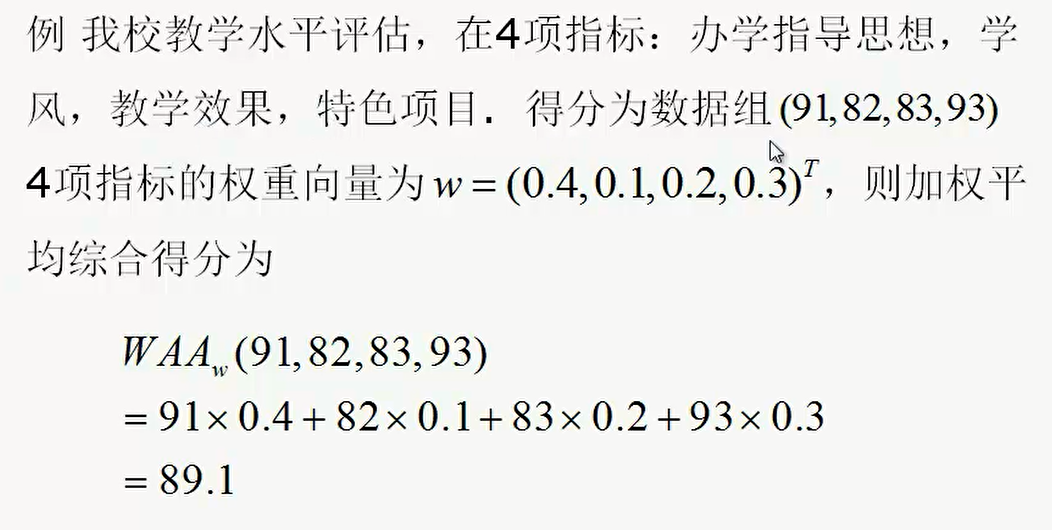## 2. 属性值归一化处理• 效益型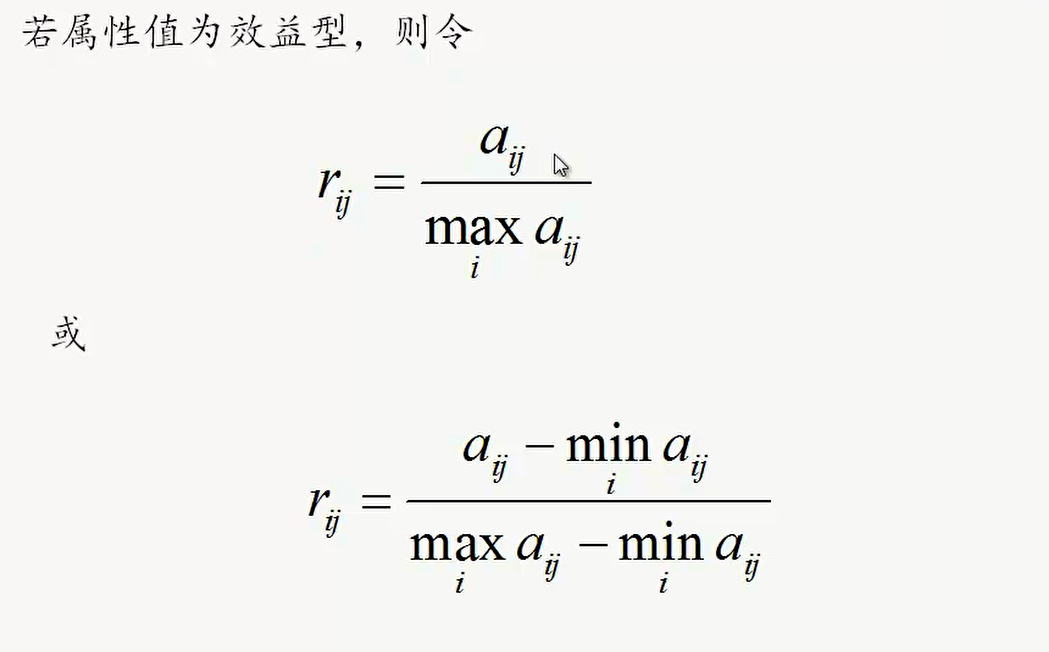• 成本型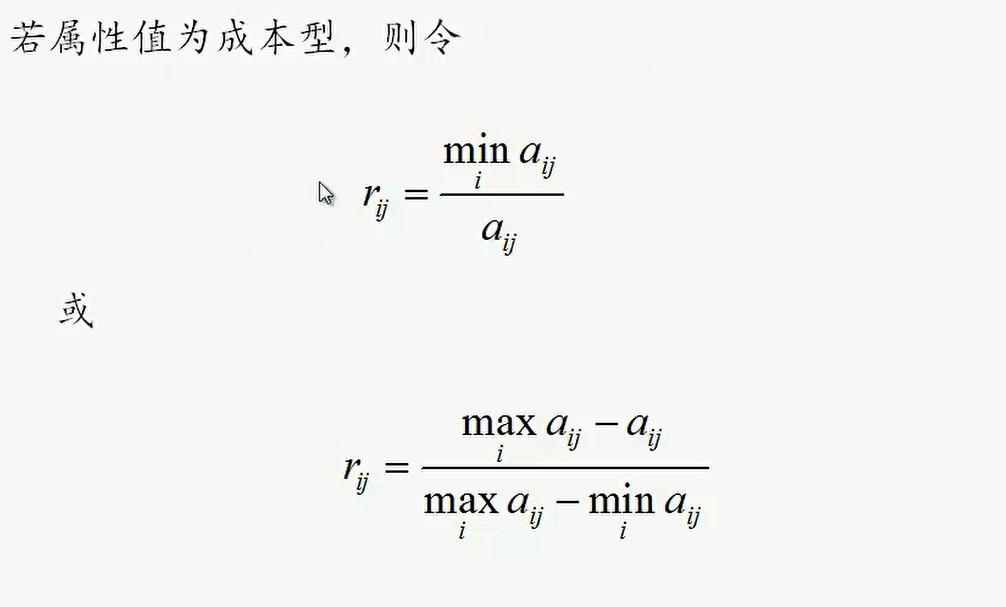• 固定型、偏离型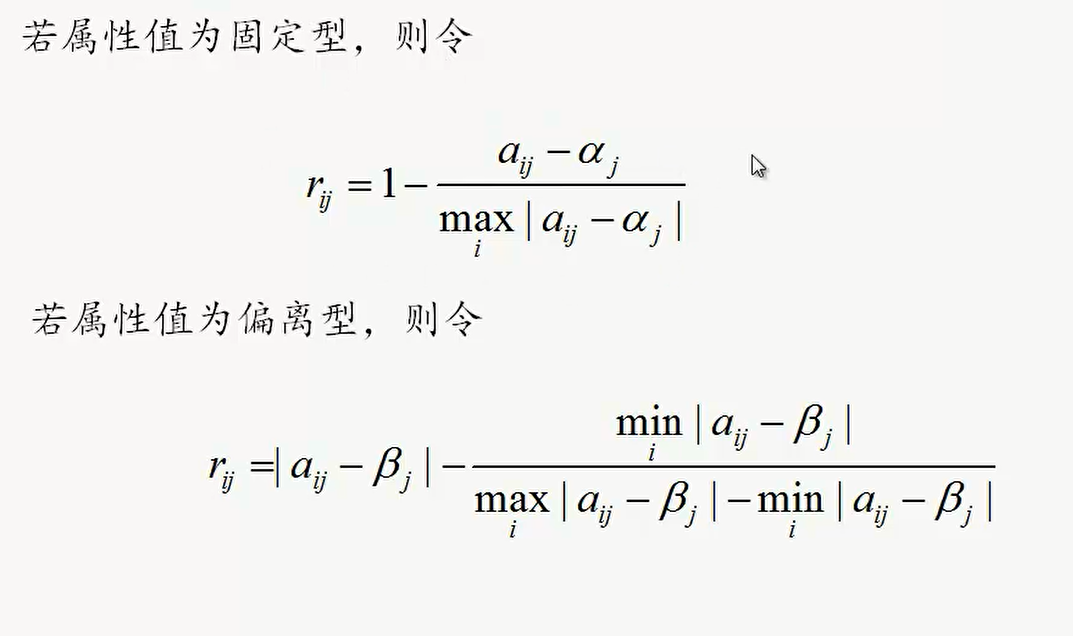• 区间型、偏离区间型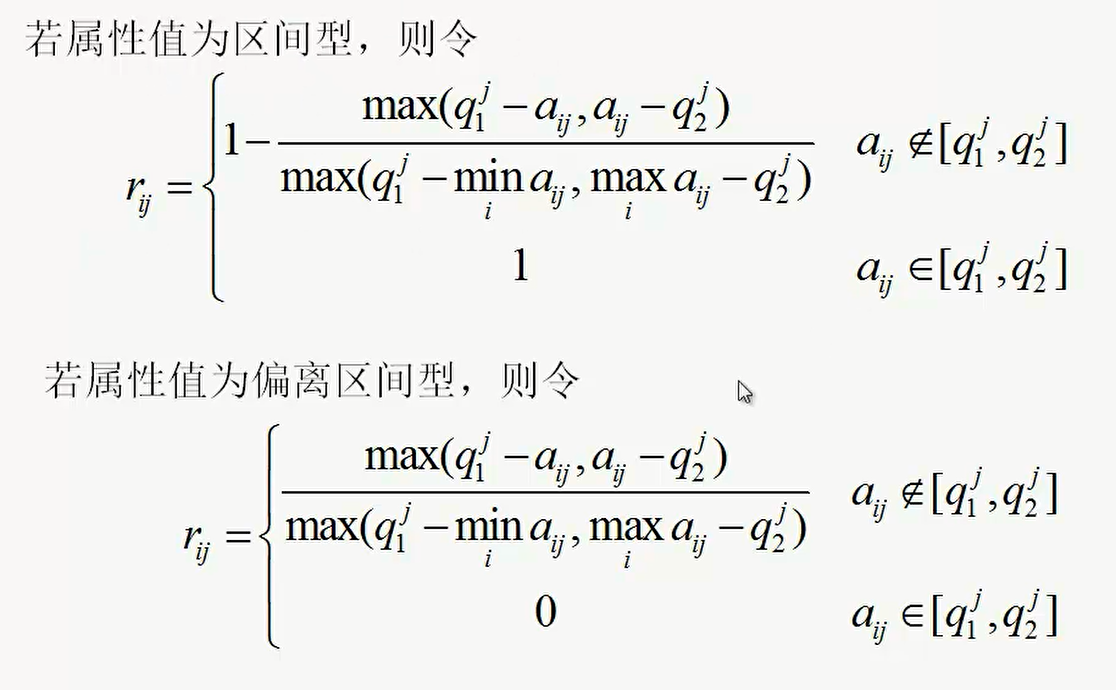# 二、适用问题

• 面临多种方案时,根据多个属性及一定的标准选择某一种方案：
• 例如：
1. 银行根据多个属性对公司进行评估

# 三、算法总结

## 1. 步骤

1. 建模构建决策矩阵
2. 属性值归一化
3. 对各个属性构造成对比较矩阵计算属性权重
4. 计算每个公司的WAA

# 四、应用场景举例（企业评估）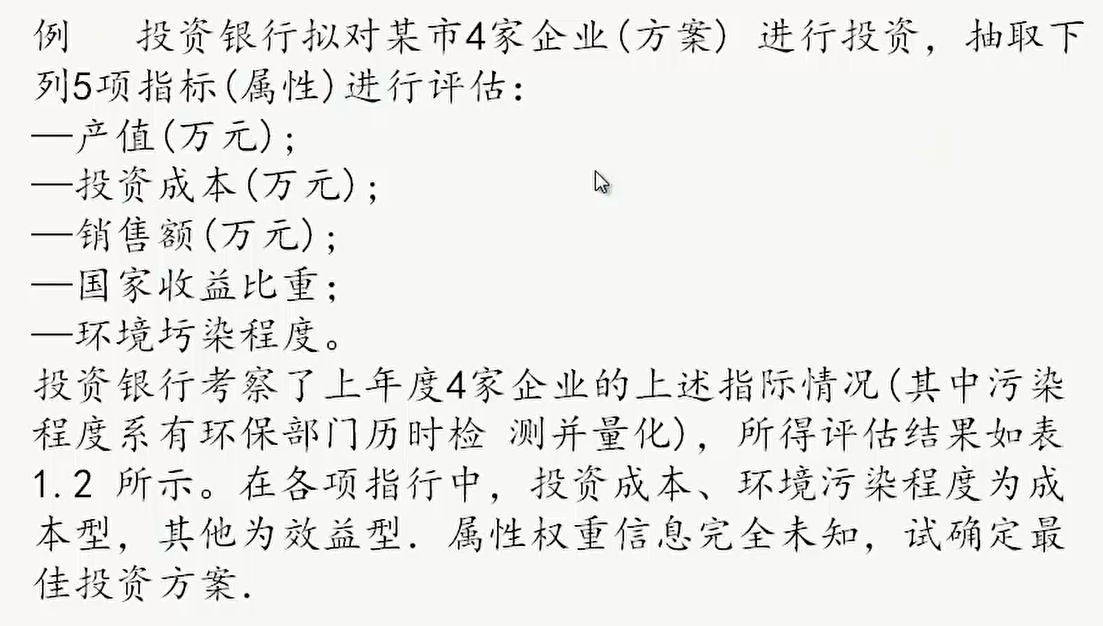## 2. 属性值归一化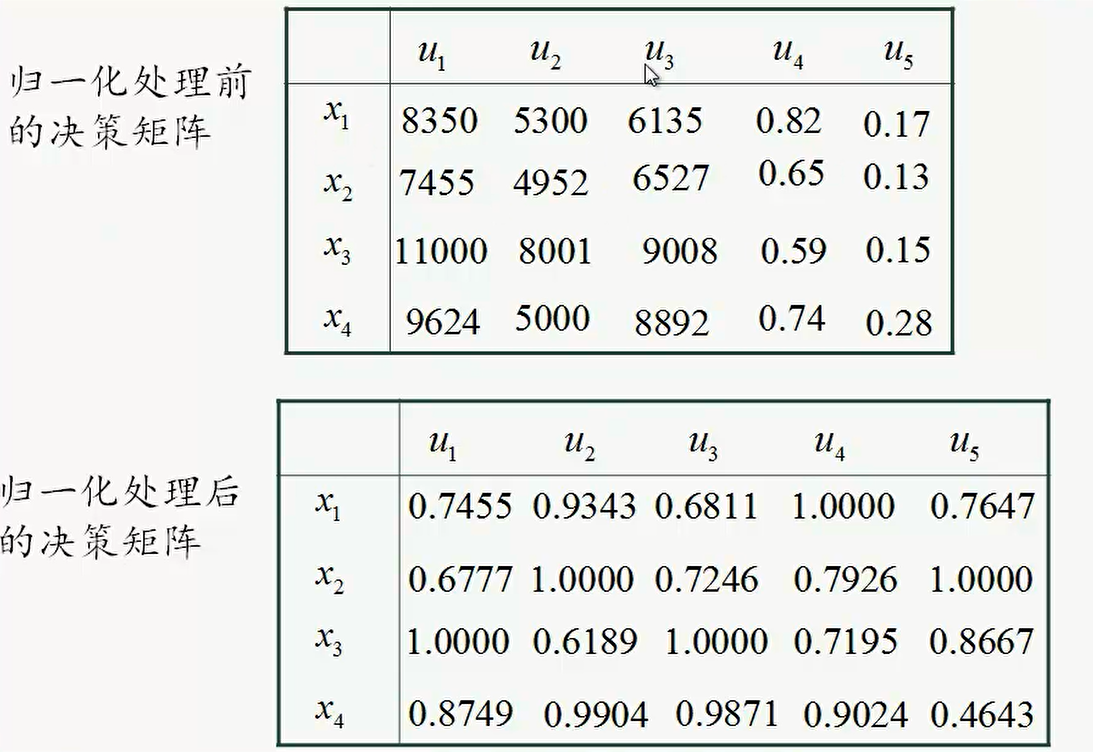## 3. 对各个属性构造成对比较矩阵计算属性权重（层次分析法）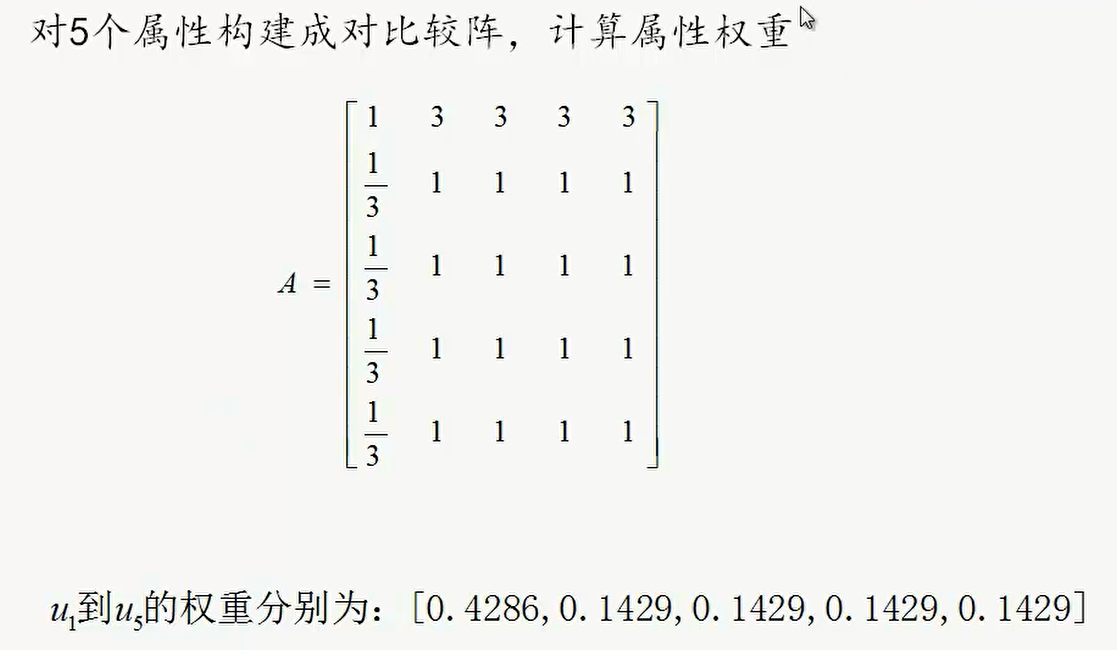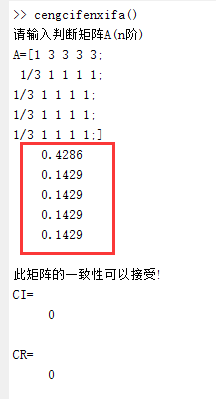## 4. 计算每个公司的WAA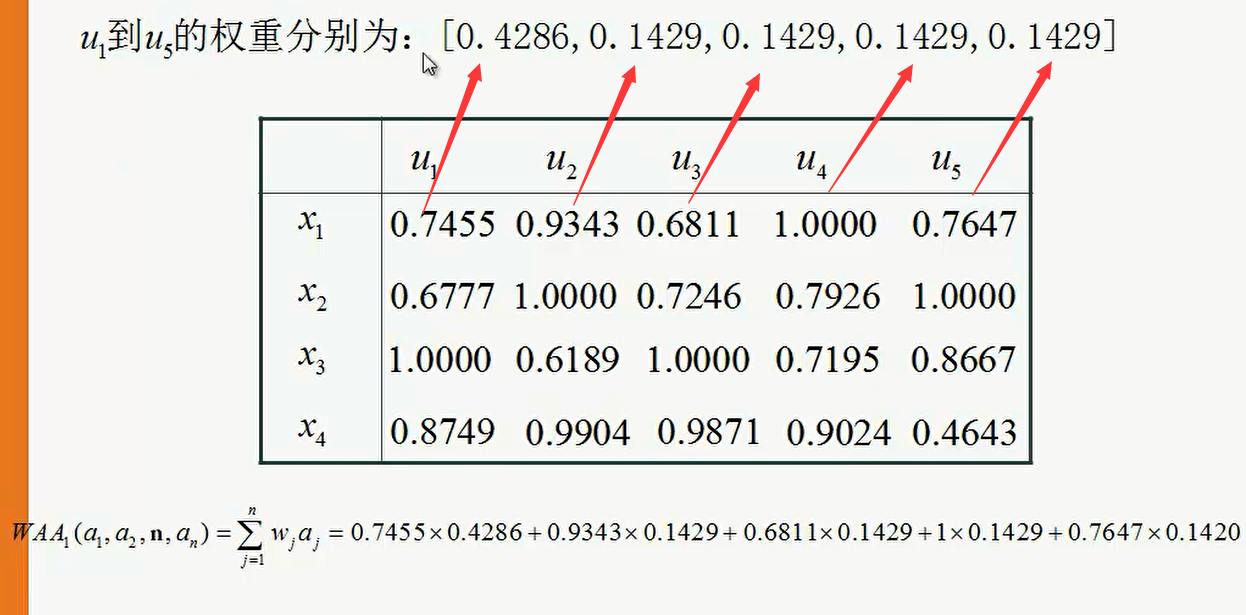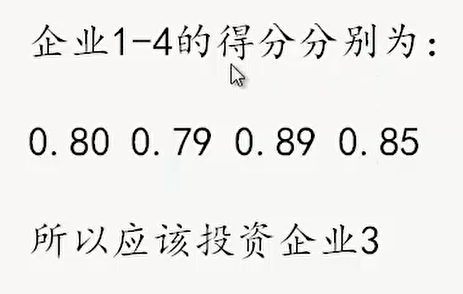# 五、MATLAB代码

disp('请输入判断矩阵A(n阶)');
A=input('A=');
[n,n]=size(A);
x=ones(n,100);
y=ones(n,100);
m=zeros(1,100);
m(1)=max(x(:,1));
y(:,1)=x(:,1);
x(:,2)=A*y(:,1);
m(2)=max(x(:,2));
y(:,2)=x(:,2)/m(2);
p=0.0001;i=2;k=abs(m(2)-m(1));
while  k>p
i=i+1;
x(:,i)=A*y(:,i-1);
m(i)=max(x(:,i));
y(:,i)=x(:,i)/m(i);
k=abs(m(i)-m(i-1));
end
a=sum(y(:,i));
w=y(:,i)/a;
t=m(i);
disp(w);
%以下是一致性检验
CI=(t-n)/(n-1);RI=[0 0 0.52 0.89 1.12 1.26 1.36 1.41 1.46 1.49 1.52 1.54 1.56 1.58 1.59];
CR=CI/RI(n);
if CR<0.10
disp('此矩阵的一致性可以接受!');
disp('CI=');disp(CI);
disp('CR=');disp(CR);
end



# 六、实际案例

[1 3 3 3 3;
1/3 1 1 1 1;
1/3 1 1 1 1;
1/3 1 1 1 1;
1/3 1 1 1 1;]


# 七、论文案例片段（待完善）

09-28
10-0832508-273686
05-07979
09-0884
07-22891
07-21
04-24点击重新获取扫码支付余额充值